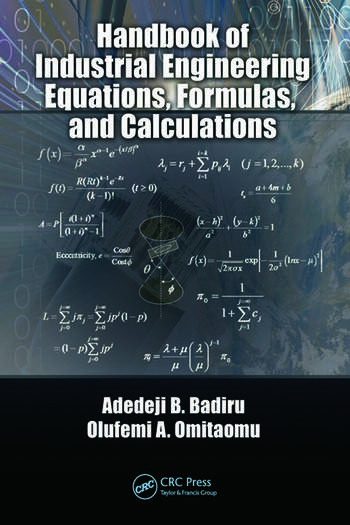Handbook of Industrial Engineering Equations, Formulas, and Calculations

1st Edition

CRC Press
Published September 17, 2010
Reference - 456 Pages - 98 B/W Illustrations
ISBN 9781420076271 - CAT# 76272
Series: Systems Innovation Book Series

USD\$185.00

FREE Standard Shipping!

Preview

Summary

The first handbook to focus exclusively on industrial engineering calculations with a correlation to applications, Handbook of Industrial Engineering Equations, Formulas, and Calculations contains a general collection of the mathematical equations often used in the practice of industrial engineering. Many books cover individual areas of engineering and some cover all areas, but none covers industrial engineering specifically, nor do they highlight topics such as project management, materials, and systems engineering from an integrated viewpoint.

Written by acclaimed researchers and authors, this concise reference marries theory and practice, making it a versatile and flexible resource. Succinctly formatted for functionality, the book presents:

• Basic Math Calculations
• Engineering Math Calculations
• Production Engineering Calculations
• Engineering Economics Calculations
• Ergonomics Calculations
• Facility Layout Calculations
• Production Sequencing and Scheduling Calculations
• Systems Engineering Calculations
• Data Engineering Calculations
• Project Engineering Calculations
• Simulation and Statistical Equations

It has been said that engineers make things while industrial engineers make things better. To make something better requires an understanding of its basic characteristics and the underlying equations and calculations that facilitate that understanding. To do this, however, you don’t have to be computational experts; you just have to know where to get the computational resources that are needed. This book elucidates the underlying equations that facilitate the understanding required to improve design processes, continuously improving the answer to the age-old question: What is the best way to do a job?